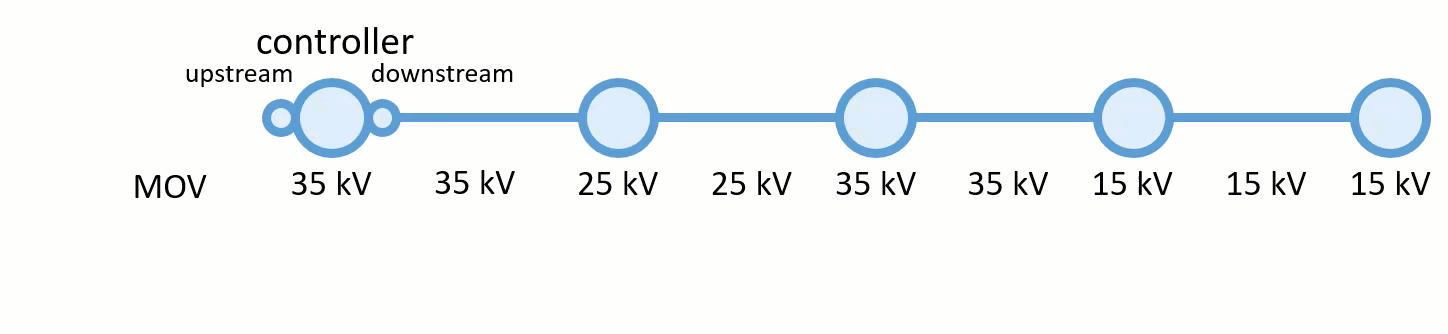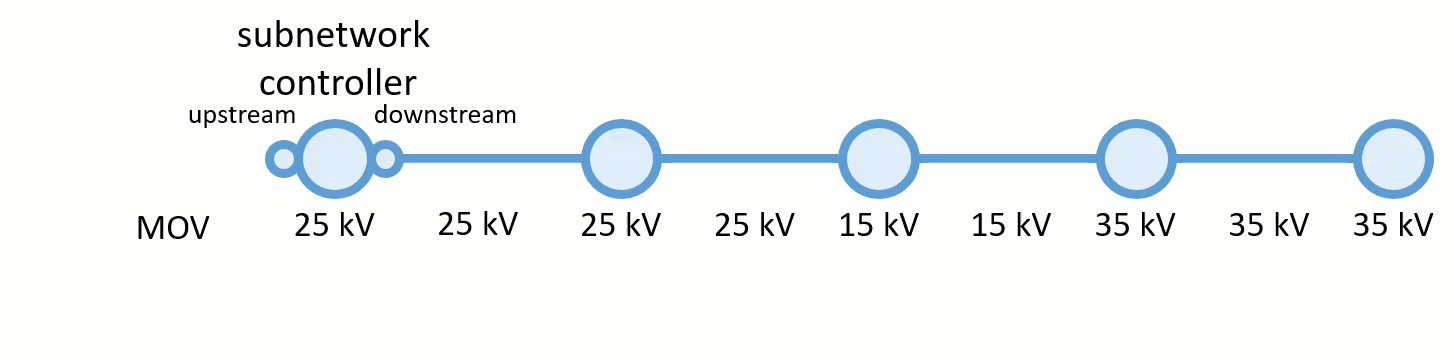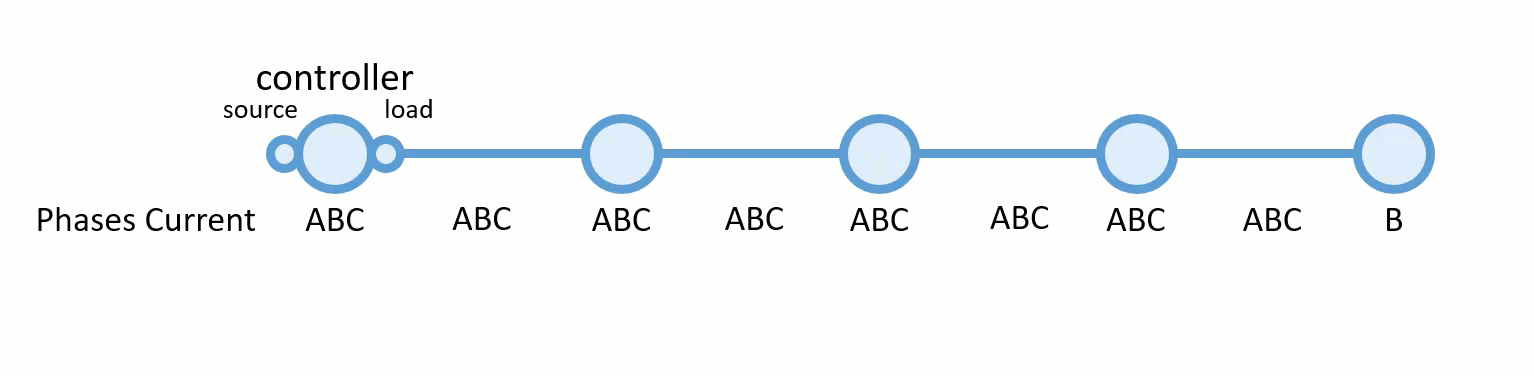# Attribute propagation

Utility networks provide an advanced functionality known as attribute propagation that is used when a subnetwork is updated, exported, or traced.

Propagators derive values from network attributes for features downstream of subnetwork controllers as the trace traverses the features. A network attribute is persisted inside the network topology when the topology is enabled or validated, and it is associated with a value stored in an attribute on a feature class.

##### Note:

If a network attribute supports null values, null values encountered during propagation are ignored and have no impact on the value being propagated.

Propagators are defined at the tier level as part of the subnetwork trace configuration on a single network attribute. This is completed by the utility network administrator during the configuration phase of a utility network using Set Subnetwork Definition or when executing . The propagators parameter is only available when using Python or creating a variable from a parameter in ModelBuilder.

Propagator configurations can be found on the Network Properties tab within the Trace Configuration column of the Tiers subsection. When a subnetwork is updated, exported, or traced, an operator is used to filter which features are considered. Several parameters are associated with a propagation configuration. To learn more, see Set Subnetwork Definition.

There are three Functions available for propagating a network attribute value:

• Propagated_MIN
• Propagated_MAX
• Propagated_BITWISE_AND
Propagated_MIN and Propagated_MAX are used for numeric values, whereas Propagated_BITWISE_AND is used for numeric values that represent a bitset.
##### Note:

Propagation always starts from the subnetwork controller.

The following examples demonstrate source-based network scenarios; however, upstream and downstream could be reversed to apply to sink-based networks.

## Propagated_MIN

The Propagated_MIN function takes the minimum value from the two numeric values being compared. This function should be used when a numeric value on the current feature needs to remain equal to or less than the value on the previous feature.

As an example, consider a downstream trace in an electric network where the network attribute MOV is assigned to the field maxoperatingvoltage. The trace begins with a MOV of 35 kV, which becomes the first propagated value. When the value of 25 kV is encountered, this minimum value is then propagated until it encounters another minimum value. This operation continues downstream, propagating the minimum value until the entire subnetwork is traced or the condition set by the operator is met.

The example function illustrated below is as follows: MOV PROPAGATED_MIN IS_GREATER_THAN 15 MAXVOLTAGEThis configuration tells the system to continue propagating while MOV remains greater than 15 kV. In this example, MAXVOLTAGE is the attribute updated during execution of Update Subnetwork, and a value of 15 kV or less would act as a barrier, terminating the trace.

## Propagated_MAX

The Propagated_MAX function takes the maximum value from the two numeric values being compared. This function should be used when a numerical value on the current feature needs to remain equal to or greater than the value on the previous feature.

Consider the same electric network where MOV is a network attribute assigned to the maxoperatingvoltage field. An engineer considering upgrading the voltage on a circuit may want to run a trace to help them understand how much equipment might need to be changed as part of the project. This could be done using a propagator to update MAXVOLTAGE along the line with a trace, as long as it did not exceed 30 kV using the PROPAGATED_MAX function with the network attribute MOV as follows: MOV PROPAGATED_MAX IS_LESS_THAN_OR_EQUAL_TO 30 MAXVOLTAGEThis configuration tells the system to continue propagating while MOV remains less than 30 kV. In this example, MAXVOLTAGE is the attribute updated during execution of Update Subnetwork, and a value greater than 30 kV would act as a barrier, terminating the trace.

## Propagated_BITWISE_AND

The Propagated_BITWISE_AND function takes the difference between two numeric values that represent a bitset. For example, in the electric domain, phase can be modeled using three bits: one for phase A, one for phase B, and one for phase C. These three bits together form a binary number 111, which when converted into decimal yields the number 7. If one of the bits is de-energized (for example, bit B), leaving the other two bits energized (for example, bits A and C), the binary number becomes 101 (the 0 in the middle indicates B is de-energized), which when converted into decimal yields the number 5.

Consider a downstream trace on an electrical network where propagation is configured to update phase, where the network attribute Phases Current is assigned to the field phasescurrent.

The source circuit breaker is ABC. When the B phase is de-energized upstream, the propagator calculates the phase value of the downstream features to de-energize phase B as well, even though the network attribute of the feature may indicate phase B. This operation continues downstream with the trace as long as the operator is true.

The example function illustrated below is as follows: Phases Current PROPAGATED_BITWISE_AND INCLUDES_ANY ABC PHASEENGIn this example, Phaseseng is the attribute updated following execution of Update Subnetwork. A line carrying phase B would be de-energized by the propagator and act as a barrier for a trace.